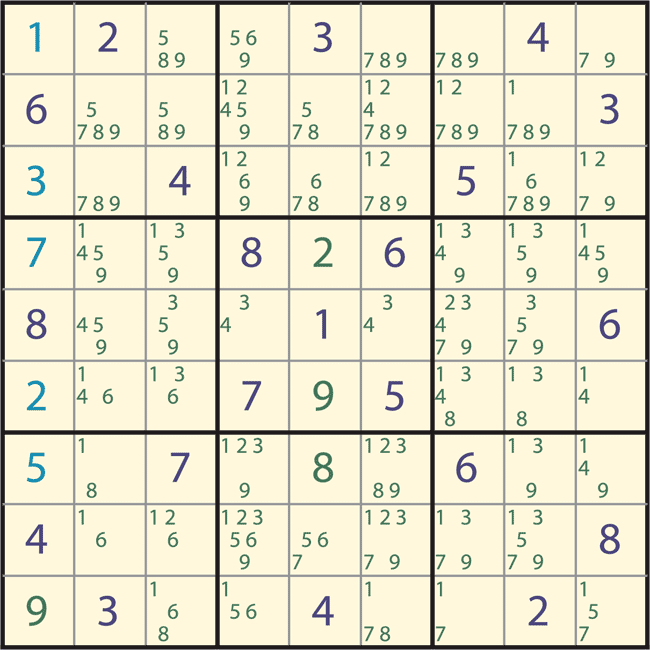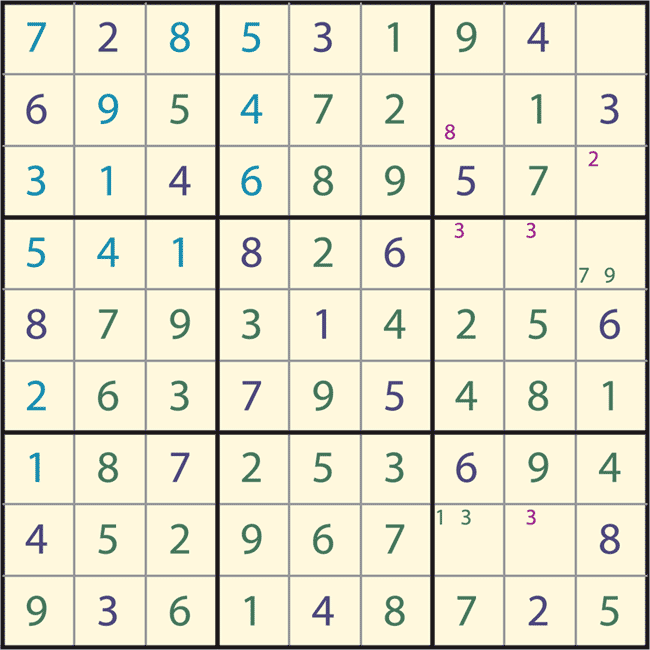Technical Articles and Newsletters

# Solving Sudoku with MATLAB

By Cleve Moler, MathWorks

Human puzzle-solvers and computer programs use very different Sudoku-solving techniques. The fascination with solving Sudoku by hand derives from the discovery and mastery of a myriad of subtle combinations and patterns that provide hints about the final solution. It is not easy to program a computer to duplicate these human pattern-recognition capabilities. For this reason, most Sudoku-solving programs take a very different approach, relying on the computer’s almost limitless capacity to carry out brute-force trial and error. That is the approach that I used for the MATLAB® program.

### The Sudoku Challenge

As you probably know, solving a Sudoku involves filling in a 9-by-9 grid so that each row, column, and major 3-by-3 block contains all the digits 1 through 9. The initial grid is populated with a few digits, known as clues. In contrast to magic squares and other numeric puzzles, no arithmetic is involved; the elements in a Sudoku grid could just as well be letters of the alphabet or any other symbols.

Figure 1 shows an initial grid. I especially like the symmetry in this example, which is due to Gordon Royle of the University of Western Australia. Figure 2 shows the solution.Figure 2. The completed puzzle. The other digits have been inserted so that each row, column, and major 3-by-3 block contains the digits 1 through 9.

### Solving Sudoku with Recursive Backtracking

Our MATLAB program uses only one pattern—singletons—together with a basic computer science technique, recursive backtracking.

To see how the program works, we can use a simpler 4-by-4 grid with 2-by-2 blocks. Such puzzles are called Shidoku instead of Sudoku because “Shi” is Japanese for “four.”

Figure 3 shows our first Shidoku puzzle. Figures 4 through 6 show its solution. In Figure 4, the possible entries, or candidates, are represented as small digits. For example, row two contains a “3” and column one contains a “1”, so the candidates in position (2,1) are “2” and “4.” Four of the cells contain only one candidate. These cells are the singletons. This first example can be solved easily by filling in the singletons. In Figure 5, we have inserted one of the singletons and recomputed the candidates. In Figure 6, we have inserted the remaining singletons as they appear to complete the solution.

An easy puzzle could be defined as one that can be solved by just inserting the singletons. Within this definition, our first example is easy, but the next example is not.

The input array for the puzzle shown in Figure 7 is generated by the MATLAB statement

` X = diag(1:4) `

Because there are no singletons in this puzzle (Figure 8), we will use recursive backtracking. We select one of the empty cells and tentatively insert one of its candidates. We have chosen to consider the cells in the order implied by MATLAB one-dimensional subscripting, X(:), and try the candidates in numerical order. So we insert a “3” in cell (2,1), creating a new puzzle (Figure 9). We then call the program recursively.

The new puzzle is easy; the result is shown in Figure 10. However, this solution depends upon the choices that we made before the recursive call. Other choices could produce different solutions. For this simple diagonal initial condition, there are two possible solutions, which happen to be matrix transposes of each other. Since the solution is not unique, the grid in Figure 7 is not a valid puzzle.

### Existence and Uniqueness

As mathematicians, we seek to prove that a solution to a problem exists, and that it is unique. With Sudoku, neither existence nor uniqueness can easily be determined from the initial clues. For example, with the puzzle shown in Figure 1, if we were to insert a “1”, “5,” or “7” in the (1,1) cell, the row, column, and block conditions would be satisfied but the resulting puzzle would have no solution. It would be very frustrating if such a puzzle were to show up in your newspaper.

Backtracking generates many impossible configurations. Our program terminates the recursion when it encounters a cell that has no candidates. Such puzzles have no solution.

Uniqueness is an elusive property. Most descriptions of Sudoku do not specify that there must be only one solution. Again, it would be frustrating to discover a solution different from the one given. Some of the puzzle-generating programs on MATLAB Central do not check uniqueness. The only way that I know to check for uniqueness is to exhaustively enumerate all possible solutions.

### The Sudoku-Solving Algorithm

Our MATLAB program involves just four steps:
1. Fill in all singletons.
2. Exit if a cell has no candidates.
3. Fill in a tentative value for an empty cell.
4. Call the program recursively.

The key internal function is `candidates`. Each empty cell starts with `z = 1:9` and uses the numeric values in the associated row, column, and block to zero elements in `z`. The nonzeros that remain are the candidates. For example, consider the (1,1) cell in Figure 1. We start with

` z = 1 2 3 4 5 6 7 8 9 `

The values in the first row change z to

` z = 1 0 0 0 5 6 7 8 9 `

Then the first column changes z to

` z = 1 0 0 0 5 0 7 0 9 `

The (1,1) block does not make any further changes, so the candidates for this cell are

` C{1,1} = [1 5 7 9] `

### A Difficult Puzzle

The puzzle shown in Figure 1 is actually very difficult to solve, either by hand or by computer. Figures 11 and 12 are snapshots of the computation. Initially, there are no singletons, so the first recursive step happens immediately. We try a “1” in the (1,1) cell. Figure 11 shows how the first column is then filled by step 22. But we’re still a long way from the solution. After 3,114 steps, the recursion puts a “5” in the (1,1) cell, and after 8,172 steps, it tries a “7.”Figure 11. The situation after just 22 steps towards the solution of Figure 1. Values colored cyan are tentative choices made by the backtracking, and values colored green are the singletons implied by those choices. The “1” is the wrong choice for the (1,1) cell. Click on image to see enlarged view.Figure 12. After 14,781 steps, we appear to be close to a solution, but it is impossible to continue because there are no candidates for cell (1,9). The “7” is the wrong choice for the (1,1) cell. Click on image to see enlarged view.

Figure 12 shows the situation after 14,781 steps. We appear to be close to a solution because 73 of the 81 cells have been assigned values. But the first row and last column, taken together, contain all the digits from 1 through 9, so there are no values left for the (1,9) cell in the upper right corner. The candidate list for this cell is empty, and the recursion terminates. Finally, after 19,229 steps, we try a “9” in the first cell. This “9” is a good idea because less than 200 steps later, after 19,422 steps, the program reaches the solution shown in Figure 2. This is many more steps than most puzzles require.

### Solving Sudoku Using Recursive Backtracking

```function X = sudoku(X)
% SUDOKU  Solve Sudoku using recursive backtracking.
%   sudoku(X), expects a 9-by-9 array X.
% Fill in all “singletons”.
% C is a cell array of candidate vectors for each cell.
% s is the first cell, if any, with one candidate.
% e is the first cell, if any, with no candidates.
[C,s,e] = candidates(X);
while ~isempty(s) && isempty(e)
X(s) = C{s};
[C,s,e] = candidates(X);
end
% Return for impossible puzzles.
if ~isempty(e)
return
end
% Recursive backtracking.
if any(X(:) == 0)
Y = X;
z = find(X(:) == 0,1);    % The first unfilled cell.
for r = [C{z}]            % Iterate over candidates.
X = Y;
X(z) = r;              % Insert a tentative value.
X = sudoku(X);         % Recursive call.
if all(X(:) > 0)       % Found a solution.
return
end
end
end
% ------------------------------
function [C,s,e] = candidates(X)
C = cell(9,9);
tri = @(k) 3*ceil(k/3-1) + (1:3);
for j = 1:9
for i = 1:9
if X(i,j)==0
z = 1:9;
z(nonzeros(X(i,:))) = 0;
z(nonzeros(X(:,j))) = 0;
z(nonzeros(X(tri(i),tri(j)))) = 0;
C{i,j} = nonzeros(z)’;
end
end
end
L = cellfun(@length,C);   % Number of candidates.
s = find(X==0 & L==1,1);
e = find(X==0 & L==0,1);
end % candidates
end % sudoku
```

### The Origins of Sudoku

Most people assume that Sudoku originated in Japan. In fact, it is an American invention. It first appeared, with the name Number Place, in Dell Puzzle Magazine in 1979. In 1984, a Japanese publisher, Nikoli, took the puzzle to Japan and gave it the name Sudoku, which is a kanji acronym for “numbers should be single, unmarried.” The Times of London began publishing the puzzle in 2004, and it was not long before it spread to the U.S. and around the world.

Published 2009 - 91789v00